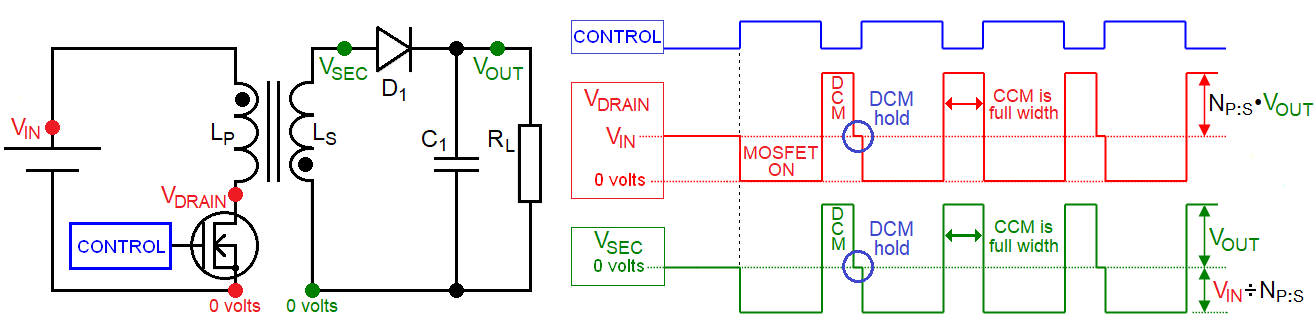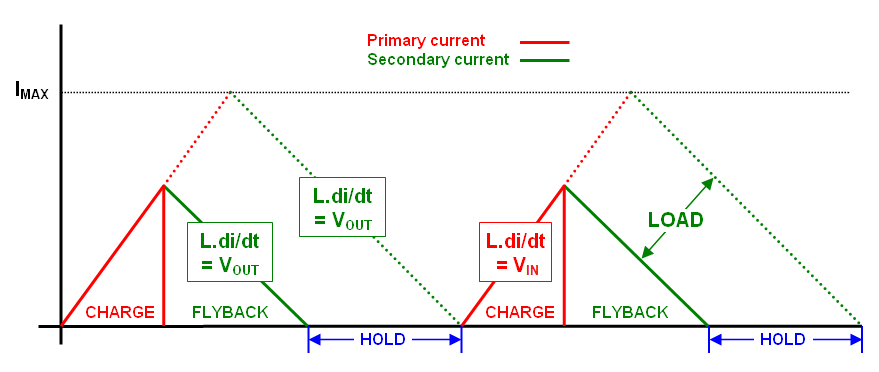A LITTLE bit of theory   In DCM (discontinuous mode), the flux will fall to zero and the core has to be designed to avoid saturation. A DCM transformer will also have a smaller primary inductance compared to a CCM design and can be simpler to control but, crossing the boundary from DCM to CCM should be avoided because instability can occur as well as core saturation.Charging When the MOSFET activates, $$V_{BUS}$$ is applied to the primary winding. The anode of $$D_1$$ becomes negative and no current passes into $$C_1$$ or $$R_L$$. Primary current ramps up at a rate defined by $$V_{BUS}$$ and $$L_P$$: -   $$\dfrac{di}{dt} = \dfrac{V_{BUS}}{L_P}$$   Flyback When the MOSFET turns off, "flyback" occurs and, the secondary voltage reverses polarity and pushes current through $$D_1$$ into $$C_1$$ and $$R_L$$. With N (the turns ratio) at 1:1, the primary current seen at the end of the charge period becomes the initial secondary current at the start of the "flyback" period. Secondary current ramps linearly down to zero amps.   The rate at which this current ramps to zero dictates the output voltage seen across the load (assuming $$D_1$$ is ideal).   Some time later, the charging process restarts and current ramps up in the primary once more. So, in a DCM design there are three phases: charging, flyback and hold: -Hold The "hold" period has a variable length because, if the load is light, the amount of energy transferred during flyback has to match the energy requirements of the load in order to keep $$V_{OUT}$$ at a regulated value. This is important to remember in the DCM design - on very light loads the charge time can be almost zero.   $$V_{BUS}$$ range This is what happens when $$V_{BUS}$$ has a range of values: -The above is an example when the $$V_{BUS}$$ range varies by 3:1. The limits would likely attract duty cycles either side of 50% such as 25% and 75%. For a $$V_{BUS}$$ range of 2 to 1, D would typically be at 50% for the approximate midpoint of $$V_{BUS}$$ hence, 100 volts would attract a duty of 66.7% and 200 volts would have D = 33.3% for example.   DCM / CCM Boundary At the boundary we can mathematically link the lowest value of $$V_{BUS}$$, $$V_{OUT}$$, the overall switching period and the energy transfer per cycle. This is because the hold period is zero. We choose $$L_P$$ to achieve a primary current of IMAX when D is at the maximum value (around 70% typically).   However, a value we cannot yet control is $$V_{OUT}$$. For a given charge-time (at the boundary), the slope of the green-line above can only be one value. However, we know that we can change the turns ratio to reduce $$V_{OUT}$$ and this will be discussed in secondary winding.   So, using simple trigonometry, we can determine the green-line slope. Below is a simple interactive representation of how $$V_{BUS}$$ and D will dictate the value of $$V_{OUT}$$ at this boundary point: -   Your browser does not support inline frames or is currently configured not to display inline frames.   Primary Flyback Voltage The "natural" value for $$V_{OUT}$$ is the slope of the green-line hence, due to 1:1 transformer action, the primary induced voltage is the same. This means that the MOSFET drain voltage is $$V_{BUS}+ V_{OUT}$$ (ignoring leakage inductance). And here's where some problems can occur.   Consider a 1:1 transformer design using a particularly light load in order to obtain a high slope green-line (a high $$V_{OUT}$$). Let's say $$V_{OUT}$$ was 500 volts. If $$V_{BUS}$$ was 100 volts then, during flyback, the MOSFET drain voltage would be 600 volts. Alternatively, if you designed the turns ratio to be 1:5  then the MOSFET drain flyback voltage would only be 200 volts (ignoring leakage inductance).   Primary Leakage Inductance This is usually a small percentage of the main primary inductance but it has a bite. When the MOSFET goes open circuit, in addition to the "desired" flyback voltage, the energy stored in the leakage inductance generates a large "spike". This has to be suppressed or damage to the MOSFET from over-voltage may occur.   So, using the 1:1 example above where $$V_{OUT}$$ was 500 volts, leakage inductance could produce a spike of several hundred volts on top of the primary flyback voltage of 600 volts. The suppression you would choose depends on many factors but it has to allow the 600 volts to "exist" or else flyback power efficiency could be severely diminished.   Candidate transformer    The Ferroxcube E38 3F3 core-set previously considered (in the ferrites section) has these characteristics: -   $$A_L$$ with a 1.1 mm air gap is 250 nH hence, with 64 turns, the inductance is 1024 µH The H-field to achieve a $$B_{max}$$ of 200 mT (due to $$\mu$$ reducing from 1520 to 45) is 777 At/m With 64 turns and an $$\ell_e$$ of 43.7 mm, IMAX is 777 x 0.0437 ÷ 64 = 0.531 amps. Operating at A switching frequency of 100 kHz (a period of 10 $$\mu$$s)    Requirements summary: - DC bus maximum = 200 volts DC bus minimum = 100 volts Primary inductance = 1024 µH Maximum primary current = 0.531 amps Using  $$V_{BUS} = L_P\dfrac{di}{dt}$$,    we can calculate the time taken (dt) for the current to rise from zero to 0.531 amps (di)   For a bus of 100 volts, dt = 1024 µH x 0.531 amps ÷ 100 volts = 5.4374 µs or a D of 0.54374 at 100 kHz. The time remaining to complete the switching cycle is 4.5626 µs and, given that the current falls to zero in this flyback period, we can calculate $$V_{OUT}$$ as: -   1024 µH x 0.531 amps ÷ 4.5626 µs = 119.17 volts   With a $$V_{BUS}$$ of 200 volts, D reduces to 50% of 0.54374: -We can also calculate the energy transfer per cycle. From this we can determine the maximum output power: -   $$W=½\cdot L_P\cdot I_{MAX}^2$$ = 144.36 µJ   $$P = W\cdot F_{sw}$$ = 14.44 watts     At higher frequencies, $$L_P$$ must reduce or we won't get nearly enough primary current. Quartering $$L_P$$ means halving the turns and, the benefit of halving the turns is that IMAX can double to 1.062 amps (without exceeding a flux density of 200 mT).   The rate at which current rises now quadruples but, because IMAX has doubled, the charge period dt becomes 2.7187 µs when $$V_{BUS}$$  is 100 volts. At this voltage, we want dt to be about 66.7% of the switching period thus, $$F_{SW}$$ = 254.42 kHz.   250 kHz seems a nice round number to use: -The flyback time period (1.2813 µs = 4 µs - 2.7187 µs) determines the "natural" output voltage when N = 1: -   256 µH x 1.062 amps ÷ 1.2813 µs = 212.18 volts   Using the energy and power calculations above, we get: -   $$W=½\cdot L_P\cdot I_{MAX}^2$$ = 144.36 µJ   $$P = W\cdot F_{sw}$$ = 36.09 watts   minimum loading is required    A flyback converter stores energy and releases it each switching cycle. Given that this is done many times per second, it should be regarded as a power regulator. It isn't a voltage regulator until you apply a control loop around the switching circuit that modifies the duty cycle to keep the output voltage regulated. This is why it is important to consider the light load scenario and the minimum duty cycle of the switching device. For instance, if the minimum duty cycle is 5%, there will always be some small amount of energy presented to the output each cycle AND, to prevent the output voltage rising continually, there must be a minimal load arrangement that consumes this small amount of energy per cycle.   With a maximum DC bus voltage of 200 volts and a 5% duty (a time period of 200 ns at 250 kHz), the current can reach 156 mA.   Using the energy formula from above above yields 3.13 µJ. At a switching frequency of 250 kHz this is a power of 781 mW. This power must be consumed by losses and the secondary load or else the output voltage will rise until that power is consumed. Using a 2:1 step down turns ratio, a $$V_{BUS}$$ of 200 volts and a switching frequency of 250 kHz we will see this: -The primary current and duty cycle remain as before but the secondary inductance has quartered and, because of this, the secondary current peak has doubled. The secondary L•di/dt has therefore halved and hence $$V_{OUT}$$ also halves. Firstly you have to consider the peak primary current and the energy contained in the inductor: $$½\cdot L_P\cdot I_{max}^2$$   Using V = L•di/dt and recognizing that at $$I_{max}$$, dt  = D ÷ Fsw (where Fsw is the switching frequency) we can say: -    $$I_{max}=\dfrac{V_{BUS}\cdot D}{L_P\cdot F_{sw}}$$   So, substituting $$I_{max}$$ into the well-known inductor energy formula we get: -   $$W=\dfrac{V_{BUS}^2\cdot D^2}{2\cdot L_P\cdot F_{sw}^2}$$   From this we can say that power in/out is: -   $$P=\dfrac{V_{BUS}^2\cdot D^2}{2\cdot L_P\cdot F_{sw}}$$   Hence,    $$\dfrac{V_{OUT}^2}{R_L}=\dfrac{V_{BUS}^2\cdot D^2}{2\cdot L_P\cdot F_{sw}}$$   And therefore,  $$\dfrac{V_{OUT}}{V_{BUS}}=\sqrt{\dfrac{R_L\cdot D^2}{2\cdot L_P\cdot F_{sw}}}$$   Taking into account that $$L_S = L_P / N^2$$ we can say that the transfer function is: -   $$\dfrac{V_{OUT}}{V_{BUS}}=\dfrac{D}{N}\cdot \sqrt{\dfrac{R_L}{2\cdot L_S\cdot F_{sw}}}$$   This means that the boundary condition is met when: -   $$\dfrac{1}{N}\cdot \dfrac{D}{1-D} = \dfrac{D}{N}\cdot \sqrt{\dfrac{R_L}{2\cdot L_S\cdot F_{sw}}}$$   Therefore,   $$D = 1-\sqrt{\dfrac{2\cdot L_S\cdot F_{sw}}{R_L}}$$ In the process of writing about the DCM flyback converter it was hoped to provide an example of where CCM has a tangible theoretical benefit. For a given primary inductance and switching frequency, DCM appears to outshine CCM in all but one area.   In CCM, the average primary current automatically "finds" a level that keeps $$V_{OUT}$$ constant across the range of permitted loads. Consider this: With a 1:1 transformer, the primary current rises from A to B in a time period defined by D hence, the secondary current falls from B to A in a time period defined by 1-D. Therefore, D defines the output voltage and, the average current in the primary naturally adjusts to the load's power requirements.   So, considering the following two scenarios running at 250 kHz with $$V_{BUS}$$ set to 100 volts and duty cycle at 34%: -CCM appears to provide better $$V_{OUT}$$ regulation over a range of output loads. This may be of benefit when multiple secondary windings are required.   Power Output During the charge cycle of Scenario A the average current is ½ x 0.531 amps = 0.2655 amps and this is further averaged across the whole switching period by D. The average current is therefore 90.23 mA. The power in/out is therefore 9.023 watts.   For Scenario B (full load), the charge period average current is 0.7964 amps reducing to an average of 0.2707 amps over the whole cycle. This is a power of 27.07 watts.   The power figure for DCM operating at 250 kHz was 36.09 watts so CCM operation is somewhat constrained. CCM holds on to energy in the transformer so if the maximum current is the same for DCM and CCM, CCM can never deliver the same power unless the switching frequency is higher.

Ω σ μ τ ω β δ η θ λ π ζ ∞ ° √ Δ ∂ ∫ Ʃ ± ≈ ≠ µ Ω ± ÷ Θ « » ≦ ≧ Σ Φ ε • θ λ ρ ω κ ² ³ ½ ¼ ¾ ° ∞ ∫≈ ≠ ≡ Ξ ∑ π Π © ®